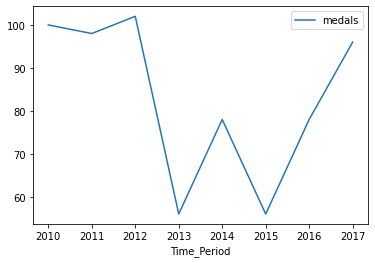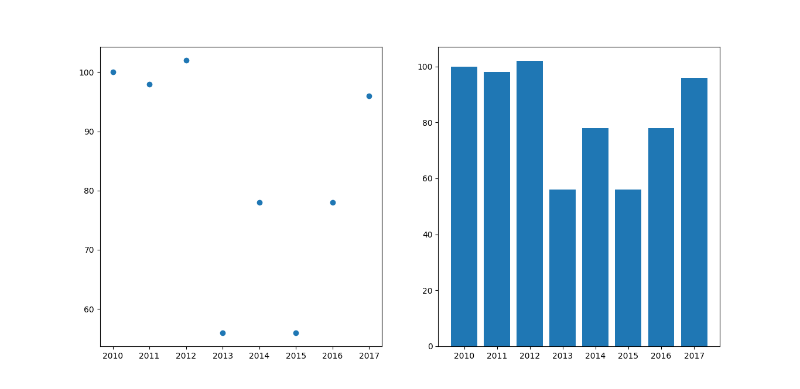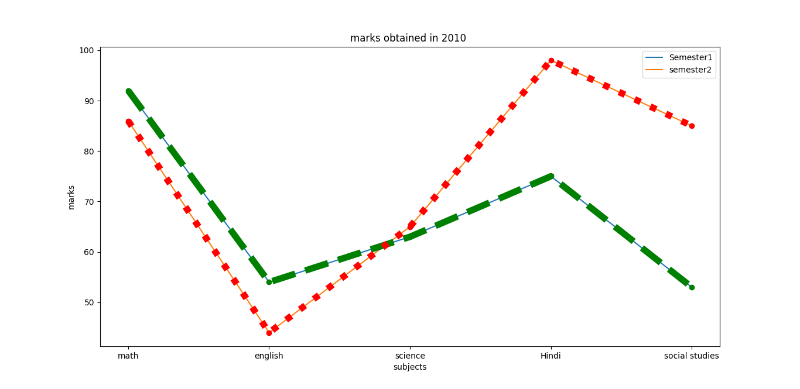Open in App
Not now

# Plotting a trend graph in Python

• Last Updated : 21 Apr, 2021

Prerequisites: Matplotlib

A trend Graph is a graph that is used to show the trends data over a period of time. It describes a functional representation of two variables (x , y). In which the x is the time-dependent variable whereas y is the collected data.  The graph can be in shown any form that can be via line chart, Histograms, scatter plot, bar chart, and pie-chart. In python, we can plot these trend graphs by using matplotlib.pyplot library. It is used for plotting a figure for the given data.

The task is simple and straightforward, for plotting any graph we must suffice the basic data requirement after this determine the values of x over the period of time and data collected for y. Plot the graphs for the above-given data.

Given below are various implementations to depict the same:

Example 1:

## Python3

 `# import all the libraries``import` `numpy as np``import` `pandas as pd``import` `matplotlib.pyplot as plt`` ` `# create a dataframe``Sports ``=` `{``    ``"medals"``: [``100``, ``98``, ``102``, ``56``, ``78``, ``56``, ``78``, ``96``],``    ``"Time_Period"``: [``2010``, ``2011``, ``2012``, ``2013``, ``2014``, ``2015``, ``2016``, ``2017``]``}`` ` `df ``=` `pd.DataFrame(Sports)``print``(df)`` ` `# to plot the graph``df.plot(x``=``"Time_Period"``, y``=``"medals"``, kind``=``"line"``)``plt.show()`

Output:

```   medals  Time_Period
0     100         2010
1      98         2011
2     102         2012
3      56         2013
4      78         2014
5      56         2015
6      78         2016
7      96         2017```Example 2: Using the above data we would plot the scatter and bar graph.

## Python3

 `# import all the libraries``import` `numpy as np``import` `pandas as pd``import` `matplotlib.pyplot as plt`` ` `# create a dataframe``Sports ``=` `{``    ``"medals"``: [``100``, ``98``, ``102``, ``56``, ``78``, ``56``,``               ``78``, ``96``],``   ` `    ``"Time_Period"``: [``2010``, ``2011``, ``2012``, ``2013``,``                    ``2014``, ``2015``, ``2016``, ``2017``]``}``df ``=` `pd.DataFrame(Sports)``print``(df)`` ` ` ` `# to plot the graph``# subplot (rowno,columno,position) is used``# to plot in a single frame.``# to plot the scatter graph ,write kind= scatter``df.plot(x``=``"Time_Period"``, y``=``"medals"``, kind``=``"scatter"``)``plt.title(``"scatter chart"``)``plt.subplot(``1``, ``1``, ``1``)`` ` ` ` `# to Plot the graph in Bar chart``df.plot(x``=``"Time_Period"``, y``=``"medals"``, kind``=``"bar"``)``plt.title(``"bar"``)``plt.subplot(``1``, ``1``, ``2``)`` ` `plt.show()`

Output:Example 3: student getting marks in 2010.

## Python3

 `# import the library``import` `matplotlib.pyplot as plt`` ` ` ` `# Creation of Data``x1 ``=` `[``'math'``, ``'english'``, ``'science'``, ``'Hindi'``, ``'social studies'``]``y1 ``=` `[``92``, ``54``, ``63``, ``75``, ``53``]``y2 ``=` `[``86``, ``44``, ``65``, ``98``, ``85``]`` ` `# Plotting the Data``plt.plot(x1, y1, label``=``'Semester1'``)``plt.plot(x1, y2, label``=``'semester2'``)`` ` `plt.xlabel(``'subjects'``)``plt.ylabel(``'marks'``)``plt.title(``"marks obtained in 2010"``)`` ` `plt.plot(y1, ``'o:g'``, linestyle``=``'--'``, linewidth``=``'8'``)``plt.plot(y2, ``'o:g'``, linestyle``=``':'``, linewidth``=``'8'``)`` ` `plt.legend()`

Output:My Personal Notes arrow_drop_up